# Polygon Coordinates and Areas

We’ve been collecting techniques for finding areas of polygons, mostly using their side lengths. We started with triangles (Heron’s formula), then quadrilaterals (Bretschneider’s formula and Brahmagupta’s formula), and the fact that the largest possible area is attained when the vertices lie on a circle. We’ll look at one more way to find area, using coordinates of vertices, before concluding with the most practical application of all these ideas: finding the area of a plot of land.

## Area of a polygon from coordinates

There are several ways to express the formula we’re interested in; I’ll introduce a couple of them, and then show a proof or two. First consider this question from 2002:

Quadrilateral Area

Given the (x,y) coordinates of four points, is there a simple formula to compute the area of the quadrilateral with corners on these 4 points?

Doctor Tom responded with the formula, which applies to any polygon, not just a quadrilateral:

Yes.

If the points are (x1, y1), (x2, y2), ..., (x4, y4), then here's the formula:

2A = (x1y2 - x2y1) + (x2y3 - x3y2) + (x3y4 - x4y3) + (x4y1 - x1y4)

(Notice the formula is for 2 times the area - to get the area, calculate the number on the right and divide by 2.)

Also, the number you get will depend on whether you go clockwise or counter-clockwise around the quadrilateral. One direction will give the negative of the other, so to get the actual area, find the area as above and then take the absolute value.

If the four points connecting 1 to 2 to 3 to 4 to 1 have lines that cross each other, the answer is meaningless - they have to form a simple region with an inside and an outside and no crossing lines.  The formula does work for non-convex quadrilaterals, however.  "Non-convex" means that there can be "indentations."

Also, I hope that the pattern of the terms above is obvious. The same formula (but with more or fewer terms) will work for any simple polygon, from triangle on up. (A "simple" polygon is one whose edges do not cross each other.)

The formula for a quadrilateral, then, is $$K = \frac{1}{2}\left|(x_1y_2 – x_2y_1) + (x_2y_3 – x_3y_2) + (x_3y_4 – x_4y_3) + (x_4y_1 – x_1y_4)\right|.$$ For the general case with n sides, we can write it as $$K = \frac{1}{2}\left|(x_1y_2 – x_2y_1) + (x_2y_3 – x_3y_2) + \dots + (x_{n-1}y_n – x_ny_{n-1}) + (x_ny_1 – x_1y_n)\right|.$$

Let’s try it out for a random non-convex quadrilateral: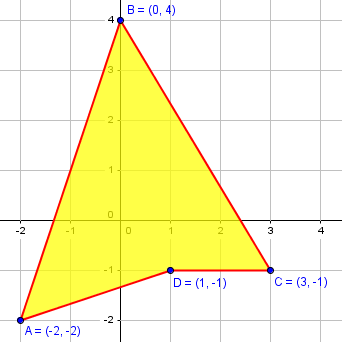Clockwise, our vertices have coordinates

• -2, -2
• 0, 4
• 3, -1
• 1, -1

The area, therefore, is $$K = \frac{1}{2}\left|(x_1y_2 – x_2y_1) + (x_2y_3 – x_3y_2) + (x_3y_4 – x_4y_3) + (x_4y_1 – x_1y_4)\right|\\ = \frac{1}{2}\left|((-2)\cdot4 – 0\cdot(-2)) + (0\cdot(-1) – 3\cdot4) + (3\cdot(-1) – 1\cdot(-1)) + (1\cdot(-2) – (-2)\cdot(-1))\right|\\ = \frac{1}{2}\left|(-8) + (-12) + (-2) + (-4)\right| = |-13| = 13.$$ The fact that we got a negative number before taking the absolute value means that we have gone clockwise around the polygon; if we had gone counterclockwise, the result would have been positive. The actual (unsigned) area is the absolute value, 13.

The formula would still work if the polygon did not contain the origin, and if the vertices did not have integer coordinates; I did that just to make the work easy.

## Determinant form (I)

The one bad thing about this formula is that, although there is a clear pattern to remember, it is a little awkward to put the right numbers in the right places. There are other ways to state it that make this easier. Consider this question from 1999:

Surveyor's Formula

I'm a student of the Politecnico di Milano (Italy) as an environmental engineer. I need a method to calculate the area of an irregular polygon in a Visual Basic program. On your website, I've read about a formula to be used in a program when I have the lengths of all the sides and some of the angles.

My problem is different: I have all the spatial topographic coordinates of the points of a drainage area that may be nonconvex.

Doctor Jerry responded with a version of the formula using determinants:

You can use the "surveyor's formula." It gives the area of any planar polygon. If the vertices are (x1,y1), (x2,y2), ..., (xn,yy), then

A = (1/2)[Det(x1,x2,y1,y2)+Det(x2,x3,y2,y3)+ ... +Det(xn,x1,yn,y1)],

where Det(a,b,c,d) = a*d-b*c.

Determinants are usually written like this: $$K = \frac{1}{2}\left(\begin{vmatrix}x_1 & x_2\\ y_1 & y_2\end{vmatrix} + \begin{vmatrix}x_2 & x_3\\ y_2 & y_3\end{vmatrix} + \dots + \begin{vmatrix}x_n & x_1\\ y_n & y_1\end{vmatrix}\right),$$ where $$\begin{vmatrix}a & b\\ c & d\end{vmatrix} = ad – bc.$$ The basic definition of the determinant is a signed sum of all products of terms in different rows and columns, which is very simple in this 2×2 case.

You can easily see that this is exactly the same formula. If you are unfamiliar with determinants, there are brief introductions to what they are here, defining them in terms of area (or volume), and also as a sum of all possible products:

Explaining the Determinant
Determinant of a Matrix

There is, of course, a lot more to say about them, including how to evaluate larger determinants.

## Shoelace Formula

But there is an even nicer way to organize the formula, which is commonly called the Shoelace Formula. It is shown in the answer to this question from 2008:

Finding the Area of an Irregular Polygon

What is the formula for finding the area of an irregular polygon?

Doctor Ali answered with some inventive terminology:

Let's say the we have an n-gon of A1-A2-A3-...-An.

Let's say that the coordinates of Ai are (Xi, Yi) and we have all the Xi's and Yi's. Then the area A is given by the 'ladder':

|x1   y1|
|x2   y2|
|x3   y3|
|   .   |
|   .   |
A = 1/2 * |   .   |
|   .   |
|   .   |
|   .   |
|xn   yn|
|x1   y1|    <-- Note that this is the first row,
repeated.

How do you evaluate the ladder?  I'll explain.

You have to multiply each number of the first column with the number in the second column of the next row.  Sum the products, and call the sum S1.

Then multiply each number of the second column with the number in the first column of the next row.  Sum the products, and call the sum S2.

Finally,

A = 1/2 * |S1 - S2|

where |x| denotes the absolute value.

You may observe that this is the same formula as before, but with all additions collected together, and all subtractions collected together. It may actually be carried out either way and still called the Shoelace Formula.

For example, consider a triangle whose vertices are at (x1,y1), (x2,y2), (x3,y3).  Here's the ladder,

|x1  y1|
|x2  y2|
|x3  y3|
|x1  y1|

here are the sums,

S1 = x1*y2 + x2*y3 + x3*y1

S2 = y1*x2 + y2*x3 + y3*x1

and here is the area:

A = 1/2 * |S1 - S2|

= 1/2 * |x1*y2 + x2*y3 + x3*y1 - y1*x2 - y2*x3 - y3*x1|

This theorem comes from "The Surveyor’s Area Formula".  The difference is that, in the main formula, the area is calculated by the sum of 2x2 determinants.  Here, I tried to simplify the formula by defining a SUPER-DETERMINANT--not a real mathematical term, I made it myself(!).

Wikipedia has an illustration that can’t be ignored, showing why it is called the Shoelace formula, and how it works: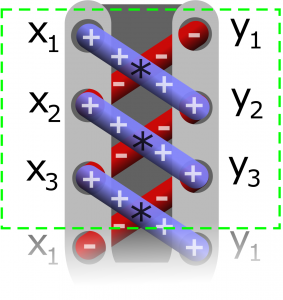## Why does it work?

As always, we have to ask why. If there isn’t a reason for it, it isn’t mathematics! This question, from 2008, is about the “atom” from which this “molecule” is built:

Geometric Proof of Area of Triangle Formula

I'm trying to prove the formula that the area of a triangle with co-ordinates (0,0),(x1,y1) and (x2,y2) is 1/2(x1y2 - x2y1) without using determinants.  Am sure I recall an elegant way to do this from when I was in school but that was 20 years ago so it escapes me now.

I seem to end up with a jumble of algebra when I try to "brute force" the proof but I'm sure there is a cleaner way.

If one vertex is (0,0), another (x1,y1) and another (x2,y2) then trying to use the 1/2 * base * height formula gets me the slope of one side = y1/x1 so slope of perpendicular is -x1/y1.  Can use this slope and the point (x2,y2) to get equation of perpendicular height line and then find point of intersection with line y = y1/x1 x by solving simultaneously.  Could then use this point and (c2,y2) in the formula for the distance between two points sqrt((x2-x1)^2 + (y2-y1)^2)) on top of this but the algebra gets pretty horrendous pretty quickly.

Do you see how this formula is one of the pieces from which the Shoelace is built? Given that it is true, the area of the polygon is just the sum of the areas of the triangles formed by each edge and the origin: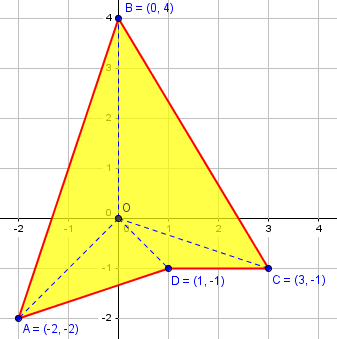If the origin is not inside the polygon, some of the areas being added will be negative, so that the total is still the polygon itself: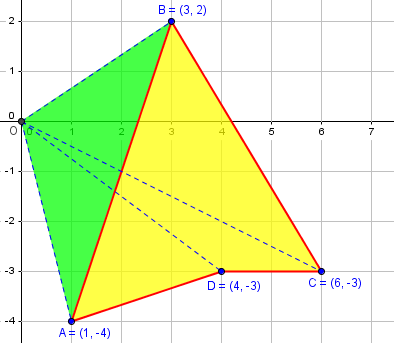We’ll be looking again at determinants soon; but Gerry wants something fundamental, and will get it. I answered:

It can be done using the vector cross-product; but maybe that's too close to determinants for your taste.

Here's a nice geometrical way, though it might take several cases to make it complete. Take this triangle:

^
|      B(x2,y2)
|     /  \
|    /     \
|   /        \
|  /           A(x1,y1)
| /       /
|/   /
O----------------->

We can add in the vertical lines to A and B:

^
|      B(x2,y2)
|     /: \
|    / :   \
|   /  :     \
|  /   :       A(x1,y1)
| /    :  /    :
|/   / :       :
O------C-------D-->

Now go around the triangle from O to A to B and back to O, looking at the "shadows" of each edge, namely triangle OAD, trapezoid ABCD, and triangle BOC.  If we take the first of these as negative (since it is below triangle OAB), and the others as positive (since they include parts of OAB), and add the areas, what do we get?

-Area(OAD) + Area(ABCD) + Area(BOC) = Area(OAB)

That is, we are subtracting the area of triangle OAD from quadrilateral ODAB which contains it, leaving only the desired triangle.  The details will vary according to the relationships of the points, but it all works out for every case.  There's probably a nicer (but perhaps hard to express) way to cover every case at once, but this satisfies me informally.

Note that the idea here is just like what I showed above for putting the triangles together, subtracting areas where the edge is “going backward”.

Now express these in terms of the coordinates:

Area(OAB) = -[x1 y1]/2 + [x1 - x2][y1 + y2]/2 + [x2 y2]/2

= [-x1 y1 + x1 y1 + x1 y2 - x2 y1 - x2 y2 + x2 y2]/2
\____________/                 \_____________/

= [x1 y2 - x2 y1]/2

This will be positive if OAB is traversed counterclockwise, and negative if it is traversed clockwise.  To be sure to get the (positive) area, take the absolute value.  And that's your formula.

The fact that the sign indicates the direction of travel relative to the origin provides a way to tell if the origin is on the “left” or “right” side of the line determined by two points. This has many uses, especially in computer graphics.

## Proof of 2×2 determinant form

This formula for the area of a triangle with one vertex at the origin can also be stated and proved in terms of vectors. See this question from 2007:

Finding Area Using a Determinant

I just can't see how this formula gives the area (determinant):

M = | 1 1|
|-1 1|

det M = 1(1) - 1(-1) = 1 + 1 = 2, which is indeed the area of the parallelogram defined by the two vectors (1,-1) and (1,1).

What are the relations in the formula?  I've tried putting them on paper numerous times, but I just can't figure it out!

To be clear, the formula for the area of the parallelogram formed by vectors $$(x_1, y_1)$$ and $$(x_2, y_2)$$ is $$K = \begin{vmatrix}x_1 & x_2\\ y_1 & y_2\end{vmatrix},$$ just as we saw as part of Doctor Jerry’s determinant form above.

This formula gives the area of a parallelogram formed by adding two vectors; the triangle we are interested in is half of that: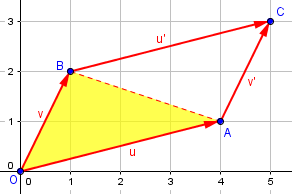In this example, the vectors are u = (4, 1) and v = (1, 2), so the parallelogram area is $$\begin{vmatrix}4 & 1\\ 1 & 2\end{vmatrix} = (4)(2) – (1)(1) = 7;$$ the triangle’s area is 3.5.

Doctor Fenton used vectors, trigonometry, geometry, and algebra to explain:

Thanks for writing to Dr. Math.  This isn't a trivial result.

This can be shown by some algebra and the dot product for vectors.  If u = (x1,y1) and v = (x2,y2) are vectors, and ||u|| represents the length of u, etc., then the dot product of u and v, u.v, is defined by

u.v = x1*x2 + y1*y2 .

Using the Law of Cosines, it can be shown that

u.v = ||u||*||v||*cos(@)

where @ is the angle between u and v.

The area of the parallelogram formed by u and v is given by

^---------------
/|              /
u  / |             /
/  |h           /
/   |           /
/@   |          /
---------------->
v
|<--->|
L

Area = h*||v||

since ||v|| is the length of the base, and h is the altitude as shown in the diagram.

Here is the picture, in relation to my vectors above: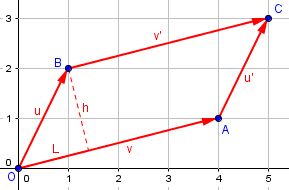If L is the length of the projection of the vector u on the vector v as shown, then

L
----- = cos(@)  ,  or L = ||u||*cos(@) .
||u||

By Pythagoras,

h^2 = ||u||^2 - L^2
= ||u||^2 - (||u||*cos(@))^2

(u.v)^2
= ||u||^2 - ||u||^2 * -----------------
||u||^2 * ||v||^2

Multiplying by ||v||^2 gives

(Area)^2 = ||u||^2 * ||v||^2 - (u.v)^2

= (x1^2 + y1^2)*(x2^2 + y2^2) - (x1*x2 + y1*y2)^2

If you simplify this expression, you can show that this equation can be written as

(Area)^2 = (x1*y2 - x2*y1)^2  ,

and the right side is the square of

det [x1 y1]
[x2 y2]   .

Another direction one could have gone is to use the vector product (cross product), whose magnitude is the area of the parallelogram.

## Area of a triangle as a determinant (II)

There is a very different-looking (but equivalent) formula for the area of a triangle, specifically, using a 3×3 determinant. Looking through our archives for mentions of it, I found at least four different orientations given: $$\frac{1}{2}\begin{vmatrix}1 & x_1 & y_1\\ 1 & x_2 & y_2\\ 1 & x_3 & y_3\end{vmatrix}$$ $$\frac{1}{2}\begin{vmatrix}x_1 & y_1 & 1\\ x_2 & y_2 & 1\\ x_3 & y_3 & 1\end{vmatrix}$$ $$\frac{1}{2}\begin{vmatrix}x_1 & x_2 & x_3\\ y_1 & y_2 & y_3\\ 1 & 1 & 1\end{vmatrix}$$

If you know about determinants, you know that these are all equivalent; the fact that we give various forms shows that the order doesn’t matter, and each of us either remembers whatever form makes sense, or just reconstructs it in a random orientation on demand!

Here is a question asking about a proof for this formula, which as you will see is really identical to the formula above:

Determinants and the Area of a Triangle

The area of a triangle having vertices (A,B), (C,D), and (E,F) is the absolute value of the determinant of M, where:

| A B 1 |
M = 1/2 | C D 1 |
| E F 1 |

How did the textbook arrive at this formula?

Many thanks for any help you can give us. Quite a few students have been asking about this.

Doctor Anthony took this:

Draw a figure with vertices (A,B), (C,D), (E,F) in the first quadrant.  For the sake of argument let (A,B) be nearest the y axis, (C,D) farthest from the y axis and (E,F) between the other two vertices and lower than either so that it is closest to the x axis.

Now draw verticals from the vertices to the x axis: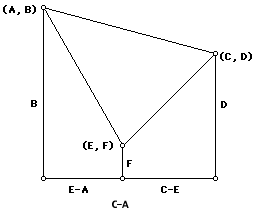The area of the triangle is found by finding the area of the largest trapezium (that with one boundary: the line joining (A,B) to (C,D)) and then subtracting two smaller trapezia, those with other two sides of the triangle as boundaries.

The large trapezium has area (1/2)(B+D)(C-A), and the smaller trapezia have areas (1/2)(B+F)(E-A) and (1/2)(F+D)(C-E).

The three regions are what Americans call trapezoids, whose area is 1/2 the sum of the bases, times the height (which here is measured horizontally).

So the area of the triangle is:

(1/2)[(B+D)(C-A) - (B+F)(E-A) - (F+D)(C-E)]

(1/2)[BC-BA+DC-DA - BE+BA-FE+FA - FC+FE-DC+DE]

(1/2)[BC - DA - BE + FA - FC + DE]

(1/2)[-AD - BE - CF + ED + FA + BC]

compared with:

|A  B  1|
(1/2)|C  D  1|  = (1/2)[AD + BE + CF - ED - FA - BC]
|E  F  1|

and apart from being opposite in sign the two expressions are the same. So the determinant gives twice the area of the triangle.

As you see, the proof for the determinant form is, ultimately, just that the determinant is the same as the Shoelace Formula. The geometrical aspect of the proof is just an extension of the proof for the triangle with a vertex at zero above.

Here is another explanation of this formula:

Finding Area Using Determinants

For a similar formula for the volume of a tetrahedron given its four vertices, see

Volume of a Tetrahedron

Next time, we’ll use these formulas and other methods to find areas of land plots.

### 3 thoughts on “Polygon Coordinates and Areas”

1.Many Thanks !

This site uses Akismet to reduce spam. Learn how your comment data is processed.